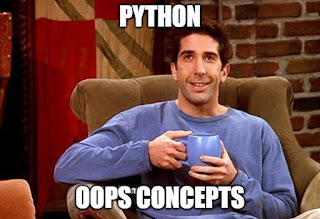## Posts

Showing posts with the label series in pandas examples

### How to use Pandas Series Method top ideasI am sharing the ideas on how to use a series constructor on a dataset using Python pandas. ...   A series is an object of Pandas that represents a one-dimensional labeled array capable of holding any data type (integers, strings, floating-point numbers, Python objects, etc.). Example of Dataframe data Dataframe data Example of Single Dimension Data A single dimension data you can derive as (index, value):  Index  Value  1  10                2  40  3  01  4  99 So, in the above "Dataframe" when you pull out the data of a single column with its index, you can say it as one-dimensional data. When one index has multiple values in brackets, you can say it as a multi-dimensional array. The example is (1, (10,20)) mySeries =  pd. Series(data, index=index) Here, pd is a Pandas object, and data refers to a Python dictionary of "ndarray" or even a scalar value. A Series is a single column of data from a DataFrame. It is a single dimension of data, composed of